# Colleges with the highest SAT scores in Rhode Island

Top 7 colleges in Rhode Island with the highest SAT scores
Looking for the colleges with the highest SAT scores in Rhode Island? Well you're in luck! We've compiled a national college database and have created a list of the top 7 universities with the highest SAT scores in Rhode Island. These are the schools whose applicants had the highest average SAT scores in Rhode Island. And, since these tests are meant to determine academic prowess, they are arguably the schools with the most academically proficient students. You could even say these are the best colleges in Rhode Island. We also include each college's ACT scores and acceptance rate so that you can see where you would have the easiest or hardest time getting in. Read on to find out more.

## Brown University SAT scores

The average SAT score for Brown University is 1485.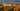The average SAT score of 1485 breaks down into:

• SAT math: 755

The average ACT score for Brown University is 34 and their acceptance rate is 7.7%.

## Rhode Island School of Design SAT scores

The average SAT score for Rhode Island School of Design is 1310.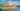The average SAT score of 1310 breaks down into:

• SAT math: 665

The average ACT score for Rhode Island School of Design is 29 and their acceptance rate is 23.9%.

## Providence College SAT scores

The average SAT score for Providence College is 1280.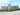The average SAT score of 1280 breaks down into:

• SAT math: 640

The average ACT score for Providence College is 29 and their acceptance rate is 47.5%.

## Bryant University SAT scores

The average SAT score for Bryant University is 1221.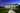The average SAT score of 1221 breaks down into:

• SAT math: 620

The average ACT score for Bryant University is 27 and their acceptance rate is 75.7%.

## Roger Williams University SAT scores

The average SAT score for Roger Williams University is 1183.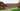The average SAT score of 1183 breaks down into:

• SAT math: 590

The average ACT score for Roger Williams University is 26 and their acceptance rate is 82.3%.

## The University of Rhode Island SAT scores

The average SAT score for The University of Rhode Island is 1179.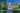The average SAT score of 1179 breaks down into:

• SAT math: 589

The average ACT score for The University of Rhode Island is 25 and their acceptance rate is 72.3%.

## Rhode Island College SAT scores

The average SAT score for Rhode Island College is 1000.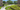The average SAT score of 1000 breaks down into:

• SAT math: 490